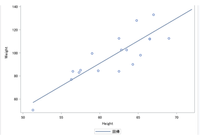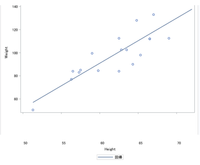## Space between tick and tick label in proc gplot

Hi,all 🙂
I'm looking for a way to add margin (space / offset) between tick and tick label with proc gplot.
Even with the method in the case of "proc sgplot", it is ok, but we are seeking information with proc gplot as much as possible.
I attach an image if what I want to do.
Those who knows, thank you for your cooperation.

``````proc sgplot data=sashelp.class;
reg x=height y=weight;
run;``````

output image of above code isAnd what I want to do is below.

Check X axis label position.Have a fine day.

1 ACCEPTED SOLUTION

Accepted SolutionsArtC
Rhodochrosite | Level 12

## Re: Space between tick and tick label in proc gplot

Here are three starter solutions.  Each could be refined depending on which way works best for you.

``````symbol v=dot color=blue;

* Solution #1;
axis1 order=(11 to 16)
minor=none
major=(height=10 color=white );
proc gplot data=sashelp.class;
plot height*age/haxis=axis1;
run;
quit;

* Solution #2;
goptions reset=axis;
axis1 style=0;
axis2 order=(40 to 80 by 10)
value=(t=1 color=white)
minor=(n=1);
proc gplot data=sashelp.class;
plot height*age/haxis=axis1
vaxis=axis2
vref=50;
run;
quit;

* Solution #3;
goptions reset=axis;
axis1 style=0;
axis2 offset=(10)
minor=(n=1);
proc gplot data=sashelp.class;
plot height*age/haxis=axis1
vaxis=axis2
vref=50;
run;
quit;

* Solution #4;
goptions reset=axis;
axis1 style=0;
axis2 style=0
offset=(10)
minor=(n=1);

data anno (keep=function xsys ysys x y line);
retain xsys ysys '2' line 1 x 11;
function='move';
y=50; output anno;
function='draw';
y=80; output anno;
run;

proc gplot data=sashelp.class
anno=anno;
plot height*age/haxis=axis1
vaxis=axis2
vref=50;
run;
quit;``````
2 REPLIES 2ArtC
Rhodochrosite | Level 12

## Re: Space between tick and tick label in proc gplot

Here are three starter solutions.  Each could be refined depending on which way works best for you.

``````symbol v=dot color=blue;

* Solution #1;
axis1 order=(11 to 16)
minor=none
major=(height=10 color=white );
proc gplot data=sashelp.class;
plot height*age/haxis=axis1;
run;
quit;

* Solution #2;
goptions reset=axis;
axis1 style=0;
axis2 order=(40 to 80 by 10)
value=(t=1 color=white)
minor=(n=1);
proc gplot data=sashelp.class;
plot height*age/haxis=axis1
vaxis=axis2
vref=50;
run;
quit;

* Solution #3;
goptions reset=axis;
axis1 style=0;
axis2 offset=(10)
minor=(n=1);
proc gplot data=sashelp.class;
plot height*age/haxis=axis1
vaxis=axis2
vref=50;
run;
quit;

* Solution #4;
goptions reset=axis;
axis1 style=0;
axis2 style=0
offset=(10)
minor=(n=1);

data anno (keep=function xsys ysys x y line);
retain xsys ysys '2' line 1 x 11;
function='move';
y=50; output anno;
function='draw';
y=80; output anno;
run;

proc gplot data=sashelp.class
anno=anno;
plot height*age/haxis=axis1
vaxis=axis2
vref=50;
run;
quit;``````

## Re: Space between tick and tick label in proc gplot

Hi,ArtC. Thank you very very much for your answering.It's so surprise that we have many ways for what I want on axis label !!
Discussion stats
• 2 replies
• 1844 views
• 2 likes
• 2 in conversation